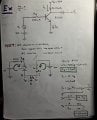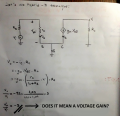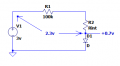# Input and Output Impedance of the Common Emitter Amplifier

#### cikalekli

Joined Dec 10, 2020
103
Hi, I just have solved one of the bjt common emitter question below.
However, I'm curious that how can I additionally find those things below:
1) Input and Output Impedance
2) AC Gain

Here is the example and my hand draw solution. However, the question only asked to find Vout/Vin...

Also, my 3rd question is, Does Vout/Vin (I calculated as -3) mean voltage gain?

Another my 4th question is Does voltage gain mean mean AC gain? Or, what is AC gain exaclty? I am not sure

I will be enormously happy if you satisfy my curiosity and show me the right way...

Also, if you have suggestions to solve this question in a more usable way, I am open to listen your suggestions.

I'm just secondary class student, that is why I try to figure out with questioning...#### ericgibbs

Joined Jan 29, 2010
16,842
hi cika.

3V source 100K in series with 0.6V drop diode, Ib = 23mA. !!!!
E

#### ericgibbs

Joined Jan 29, 2010
16,842
hi cika,
If you are calculating the input impedance of the amplifier, surely it must be a high value..
What do you think the approximate value by looking at the circuit.?
E

•cikalekli

#### cikalekli

Joined Dec 10, 2020
103
hi cika,
If you are calculating the input impedance of the amplifier, surely it must be a high value..
What do you think the approximate value by looking at the circuit.?
E
I would say just like that:
Rin = RB || rπ
= RB || (β+1) re
= (100k x 1.09k ) / (100k + 1.09k)
= 1.078247107
but I'm not quite sure.

I have found thise sheet and followed its rule. We didn't cover up to find those impedance values:
Here:

#### Attachments

• 56 KB Views: 2

#### cikalekli

Joined Dec 10, 2020
103
No no it was not an assignment.

Here I took this question from this youtube video:

I am asking how to find those impedance values I meant.

Yeah just for BJT amplifier circuit.

Thank you again. Literally I just could not understood the logic while finding impedance values.

That is why I'm just more and more I'm sinking in the mud

#### cikalekli

Joined Dec 10, 2020
103
No no it was not an assignment.

Here I took this question from this youtube video:

I am asking how to find those impedance values I meant.

Yeah just for BJT amplifier circuit.

Thank you again. Literally I just could not understood the logic while finding impedance values.

That is why I'm just more and more I'm sinking in the mud
He just did not explain how could I find those impedance values...

#### ericgibbs

Joined Jan 29, 2010
16,842
hi cika.
Checked the first part of the video,, it looks OK to me.

So lets do this step by step....

What do you calculate the Ib value? show your maths.

E

#### cikalekli

Joined Dec 10, 2020
103
hi cika.
Checked the first part of the video,, it looks OK to me.

So lets do this step by step....

What do you calculate the Ib value? show your maths.

E
Hi, now I'm in an emergency situation Eric. We need to go to the hospital for my grandmother.
Tomorrow the earlier morning, I will as soon as return to solve it step by step with your kind and wise help.

#### ericgibbs

Joined Jan 29, 2010
16,842
OK, Regards and best wishes to Grandma,,

#### Audioguru again

Joined Oct 21, 2019
5,437
The base current is not 24mA. It is 2.4uA then the collector current is correct at 2.4mA if a transistor with a beta of 100 is found.

Nobody biases a transistor like that because nobody sells a transistor with a beta of 100. A transistor part number has a range of beta maybe from 30 to 150. In your simple circuit if the beta is 30 then the transistor is almost cutoff and if the beta is 150 the transistor is saturated.

#### ericgibbs

Joined Jan 29, 2010
16,842
hi guru,
If you see the full calculations, you can see that the mA is only a typo,

The following calculations show the correct Base Impedance.

He choose a beta of 100, because that what the value given in his reference material, in order to calculate the impedances.

The transistor beta is nether 30 or 150, so what point are you trying to make.???

E

#### Audioguru again

Joined Oct 21, 2019
5,437
Many noobs make the extremely simply biased transistor circuit and are taught wrongly that the beta (of all transistors) is 100.
Then they make a simulation that works. Then they make the circuit, it does not work and come here asking what is the problem.

#### neonstrobe

Joined May 15, 2009
181
Let's be clear: are you trying to measure/calculate the input impedance of the transistor at the base, which you suggest by putting r_pi in parallel with the input resistor, or the input impedance to the amplifier, as seen by the input? Would the resistances be in parallel for this option?
For the output impedance, you need to consider the change in output voltage for a change in load impedance. That is a small signal or differential calculation, which is essentially what you did for the input. Can you do that differential?

#### cikalekli

Joined Dec 10, 2020
103
OK, Regards and best wishes to Grandma,,
Unfortunately, my grandmother has been corona and connected to the oxygen machine in the hospital for 1 month. That's why she's getting worse and worse. For this reason, yesterday I had to go to visit her with my family immediately.

#### cikalekli

Joined Dec 10, 2020
103
The base current is not 24mA. It is 2.4uA then the collector current is correct at 2.4mA if a transistor with a beta of 100 is found.

Nobody biases a transistor-like that because nobody sells a transistor with a beta of 100. A transistor part number has a range of beta that may be from 30 to 150. In your simple circuit if the beta is 30 then the transistor is almost cut off and if the beta is 150 the transistor is saturated.
My question was only understood to calculate the variables such as Vout, Vin, current, etc. You are right that is not representing a real form of the standard transistor

#### cikalekli

Joined Dec 10, 2020
103
Let's be clear: are you trying to measure/calculate the input impedance of the transistor at the base, which you suggest by putting r_pi in parallel with the input resistor, or the input impedance to the amplifier, as seen by the input? Would the resistances be in parallel for this option?
For the output impedance, you need to consider the change in output voltage for a change in load impedance. That is a small signal or differential calculation, which is essentially what you did for the input. Can you do that differential?
I was trying to figure out how I could find the input & output impedance of the amplifier.

Actually, I do know the differentiation for sure but the thing what you said sir, looked not visibly clear to me.

Could you please explain a bit specifically?

#### cikalekli

Joined Dec 10, 2020
103
hi cika.
Checked the first part of the video,, it looks OK to me.

So lets do this step by step....

What do you calculate the Ib value? show your maths.

E
Good morning Eric,

Normally initially, I always used this formula while I'm finding Ib and Ic:

Ic = Is x exp(Vbe/Vt)

and then:

Ic = β x Ib

But... particularly in this question the Is value hasn't been given at the beginning of the question.

In the video, the instructor did a different thing that I could not understand.

while he was calculating Ib, he used KVL and also added a 0.7 voltage drop factor inside his KVL equation.

Because of those both reasons, I could not understand clearly.

I hope that I've explained perspicuously

Last edited:

#### ericgibbs

Joined Jan 29, 2010
16,842
while he was calculating Ib, he used KVL and also added a 0.7 voltage drop factor inside his KVL equation.
hi cika,
Remember the Base to Emitter junction has the same characteristics as a diode
For most examples the forward voltage drop across a conducting diode is considered 0.7V.

This means that the Vsource voltage of 3V must have 0.7V subtracted ie: 3V - 0.7Vfwd = 2.3V

This means there is only a 2.3V Voltage driving current around the Base/Emitter loop.

Do you follow.?
ELast edited:

#### cikalekli

Joined Dec 10, 2020
103
hi cika,
Remember the Base to Emitter junction has the same characteristics as a diode
For most examples the forward voltage drop across a conducting diode is considered 0.7V.

This means that the Vsource voltage of 3V must have 0.7V subtracted ie: 3V - 0.7Vfwd = 2.3V

This means there is only a 2.3V Voltage driving current around the Base/Emitter loop.

Do you follow.?
E